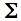Linear Correlation and Regression
Direct-EntryVersion
Fora sample of N bivariate values of X and Y, this page will calculate:T
 r = the Pearson product-moment correlation coefficient;T r2 = the coefficient of determination;T the slope of the regression line;T the Y intercept of the regression line;T the standard error of estimate;T the value of t associated with the calculated value of r, along with the corresponding one- and two-tailed probabilities;T the residual for each value of Y, calculated as residual = Y—(intercept+[slope(X)])     and:T the lower and upper limits of the .95 and .99 confidence intervals for the correlation coefficient (rho) that exists within the bivariate population from which the sample is drawn.
 The logic and computational details of correlation and regression are described in Chapter 3 of Concepts and Applications.

• ProcedureT
Enter the values of X and Y into the cells of the designated columns, beginning in the top-most cell of each column. After each entry, depressing the 'tab' key will take you down to the next cell in the column. After completion and verification of data entry, click the button labeled «Calculate». If you wish to perform another analysis with a different set of data: click the «Reset» button if the value of N for the new set of data is or smaller; click the «Reload» button if the value of N is larger than .T

Data EntryT
 Data Cells Pairs X Y Residuals

Data SummaryT
 iX = iX2i = iY = iY2i = iXY =
 X Y N Mean Variance Std.Dev. Std.Err.
 r r2 Slope YIntercept Std. Err. ofEstimate
 t df
 P one-tailed two-tailed

0.95 and 0.99 Confidence Intervals of rhoT
 Lower Limit Upper Limit 0.95 0.99

 Home Click this link only if you did not arrive here via the VassarStats main page.